# Given 2

Given g(x)=x2+x+1 where x=t2. What is g(t²)?

Result

g2 = (Correct answer is: t^4+t^2+1)### Step-by-step explanation: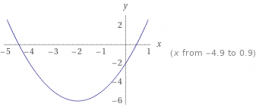Did you find an error or inaccuracy? Feel free to write us. Thank you!Tips to related online calculators

## Related math problems and questions: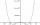By introducing a new variable solve biquadratic equation: - x 4 +277 x2 -15876=0
• Trapezoid 15Area of trapezoid is 266. What value is x if bases b1 is 2x-3, b2 is 2x+1 and height h is x+4
• Find theFind the number x, which if it increases by 2, then its square increases by 21 percent.
• Hyperbola equationFind the hyperbola equation with the center of S [0; 0], passing through the points: A [5; 3] B [8; -10]
• Unknown number 7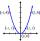Calculate unknown number whose 12th power when divided by the 9th power get a number 27 times greater than the unknown number. Determine the unknown number.
• The sumThe sum of the squares of two immediately following natural numbers is 1201. Find these numbers.
• Diamond diagonalsFind the diamond diagonal's lengths if the area is 156 cm2 and side is 13 cm long.
• Expression with powersIf x-1/x=5, find the value of x4+1/x4
• Completing squareSolve the quadratic equation: m2=4m+20 using completing the square method
• Substitution method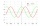Solve goniometric equation: sin4 θ - 1/cos2 θ=cos2 θ - 2
• Right triangleLegs of the right triangle are in the ratio a:b = 2:8. The hypotenuse has a length of 87 cm. Calculate the perimeter and area of the triangle.
• EQ2Solve quadratic equation: 2x²- 2(x-7)²+5x=0
• Faces diagonalsIf a cuboid's diagonals are x, y, and z (wall diagonals or three faces), then find the cuboid volume. Solve for x=1.3, y=1, z=1.2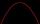Solve pure quadratic equation -5x2 +10 = 0.Solve the system of non-linear equations: 3x2-3x-y=-2 -6x2-x-y=-7How long will the pool be filled with a double supply pipe if it takes the pool to fill the first pipe by 4 hours longer and the second pipe 9 hours longer than both pipes open at the same time?Suppose the square's sides' length decreases by a 25% decrease in the content area of 28 cm2. Determine the side length of the original square.